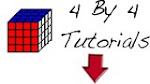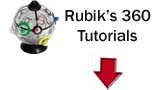How to Solve a Rubix Cube

Learn How to solve a Rubix Cube, and then start learning to become a speedcuber!

2 Look OLL

2-look OLL is simply Fridrich OLL step, but done in two stages such that the number of algorithms needed is reduced from 57 to 9.
This way of doing it is still pretty quick and you should be able to get to mid-to-high 20's averages if you use full Fridrich except that you do 2look OLL instead of the Fridrich OLL. You want to start learning all the OLL's at some point anyway though, because many of them are really easy, and they DO speed you up.

Algs:
opposite edges: F (R U R' U') F'
adjacent edges: f (R U R' U') f'
all edges: [F (R U R' U') F'] [f (R U R' U') f']

Anti-Sune: (R' U' R) U' (R' U2 R)
Sune: (R U R') U (R U2 R')
Cross Symmetric: F (R U R' U') (R U R' U') (R U R' U') F'
Cross not symmetric: (R U2) (R2 U') (R2 U') (R2 U2 R)
-----------------or: [f (R U R' U') f'] [F (R U R' U') F']
Headlights: (R2' D) (R' U2) (R D') (R' U2 R')
Chameleon: (r U R' U') (r' F R F')
Diagonal Corners orient: F' (r U R' U') r' F R